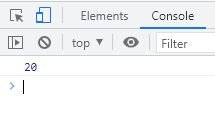# Conditional (Ternary) Operator in JavaScript

Conditional or ternary operator in JavaScript takes three operands, used as an alternative to if...else. For example:

HTML with JavaScript Code
```<!DOCTYPE html>
<html>
<body>

<script>
let x = 10, y = 20;
x>y ? console.log(x) : console.log(y);
</script>

</body>
</html>```

The snapshot given below shows the sample output produced by above JavaScript example:The same JavaScript code can also be written as:

HTML with JavaScript Code
```<!DOCTYPE html>
<html>
<body>

<p>Largest = <span id="xyz"></span></p>

<script>
let x = 10, y = 20, large;
large = x>y ? x : y;
document.getElementById("xyz").innerHTML = large;
</script>

</body>
</html>```
Output

Largest =

## JavaScript Ternary Operator (?:) Syntax

The syntax of ternary operator in JavaScript, is:

`condition ? evaluateIfTrue : evaluateIfFalse`

That is, if the condition evaluates to be True, then the whole expression replaced with evaluateIfTrue, otherwise replaced with evaluateIfFalse. For example:

`20==20 ? document.write("Both numbers are equal") : document.write("Both numbers are not equal")`

Since the condition 20==20 evaluates to be true, therefore the first expression gets executed, that is:

`document.write("Both numbers are equal")`

will be executed, and Both numbers are equal will be displayed on the output.

## Nested Ternary Operator in JavaScript

To understand nested ternary operator in JavaScript, the best and simple example that I can show you is, finding the largest of three numbers using ternary operator:

HTML with JavaScript Code
```<!DOCTYPE html>
<html>
<body>

<p>Largest = <span id="abc"></span></p>

<script>
let a = 10, b = 20, c = 30, large;
large = (a>b) ? ((b>c) ? (a) : (c>a ? c : a)) : (b>c ? b : c);
document.getElementById("abc").innerHTML = large;
</script>

</body>
</html>```
Output

Largest =

In above example, the following expression:

`(a>b) ? ((b>c) ? (a) : (c>a ? c : a)) : (b>c ? b : c);`

will be evaluated in a way that:

• First the condition (a>b) gets evaluated
• Since the condition (a>b) or (10>20) evaluates to be false
• Therefore, (b>c ? b : c) replaced the whole expression
• Now the condition b>c gets evaluated
• Since the condition b>c or 20>30 evaluates to be false
• Therefore, c replaced the whole expression
• Since the value of c is 30, therefore 30 gets initialized to large variable

JavaScript Online Test

« Previous Tutorial Next Tutorial »